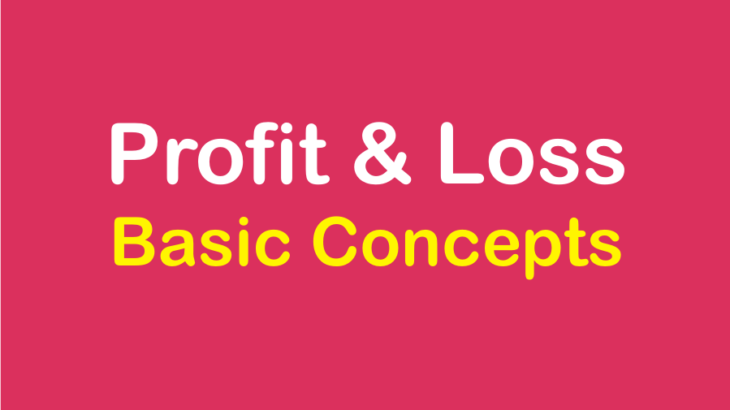# Profit and Loss: Basic Concepts and Shortcuts for CAT Exam

Profit and Loss: Basic Concepts and Shortcuts for CAT Exam
4.7 (93%) 20 vote[s]

Profit and loss based problems in CAT can easily be solved by applying the fundamental concepts of percentages. The following basic terms are widely used while solving problems on profit and loss. Hence, a clear understanding of each of these terms is essential.

### Terminologies in Profit and Loss

• Cost Price (C.P): The price at which an article is bought.
• Selling price (S.P): The price at which an article is sold.
• Profit: When an article is sold for more than the cost of the article, then we say there is a Profit or Gain.

Important: Profit or Gain = S.P — C.P

Loss: When an article is sold for less than the cost of the article,  then we say there is a Loss.
Important: Loss = C.P — S.P

Example 1: Brijesh purchased a book for Rs.1260 and sold it to Rakesh for Rs.1320. Rakesh sold it to Kishore for Rs.1400. Who gained more and by how much?
Explanation:
For Brijesh,The cost price of the book= Rs.1260.

The selling price = Rs.1320. So, the profit = Rs.1320 — Rs.1260 = Rs.60.

For Rakesh,

The cost price of the book= Rs.1320.

The selling price of the book= Rs.1400. So, the profit = Rs.1400 — Rs.1320 = Rs.80.

Clearly, Rakesh gained more than Brijesh. The difference in profits = Rs.80 — Rs.60 = Rs.20.

Thus, Rakesh gained Rs.20 more than Brijesh.

### Profit and Loss Percent

Profit or loss is also expressed as percentages of the cost price. We use the following formulas for profit or loss percent.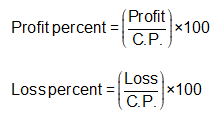Important: If the selling price of X articles is equal to the cost price of Y articles, then the net profit percentage is given by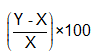.

Example 2: If the selling price of 10 articles is same as the cost price of 11 articles, find the profit or loss percent.

Explanation:

Let the cost price of 1 article be Re. 1

Therefore, the C.P. of 10 article = Rs. 10

Also, the C.P. of 11 articles = Rs. 11

Hence, Selling price (S.P.) of 10 articles = Rs. 11

Therefore, the profit percent =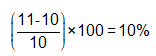Shortcut:

Here X= 10 and Y=11, therefore,  profit percent =Important: Profit and Loss are always calculated with cost price as the base.

### Selling Price Formulas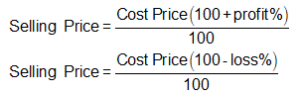Example 3: Sunil purchased apples at the rate of 4 for a rupee and sold them at the profit of 40 percent. How many Apples would a customer get for seven rupees?

Explanation:
Cost of one apple = 100 paise/4 = 25 paise.

Applying the formula for selling price.

Or, selling price of one apple =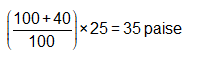Therefore, for seven rupees, the customer will get 700/35 = 20 apples.

### The concept of same selling price with profit or loss

When two products are sold at the same price (say S) in such a way that on one of the products we earn a profit and on the other we incur a loss such that the percentage of profit or loss (say x %) is the same, then for the combined transaction the net result would be a loss.

In such cases the selling price is immaterial. There is always a loss in such transactions.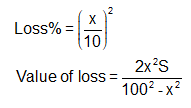Example4: Two articles are sold at Rs.198 each such that a profit of 10% is made on the first while a loss of 10% is incurred on the other. What would be the net profit/loss on the two transactions combined?
Explanation:
Article 1: Profit = 10%, Selling price = Rs.198.

⇒ Cost price = 198/1.1 = Rs.180.

Article II: Loss = 10%, Selling price = Rs.198.

⇒ Cost price = 198/0.9 = Rs.220.

Therefore, the total Cost price = Rs.180 + Rs.220 = Rs.400.

Also, Total Selling price = 2 x 198 = Rs.396.

Clearly, on the two transactions together, we have a loss of R5.400 Rs.394 = Rs.4.

Shortcut: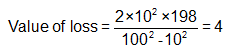Recommended: Profit and Loss Practice Problems

### Online Course for CAT 2019

Over 4000 Practice problems with video explanations in detail

Covers the entire CAT syllabus

Based on the latest CAT pattern

Online Support : live online sessions for concepts building